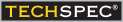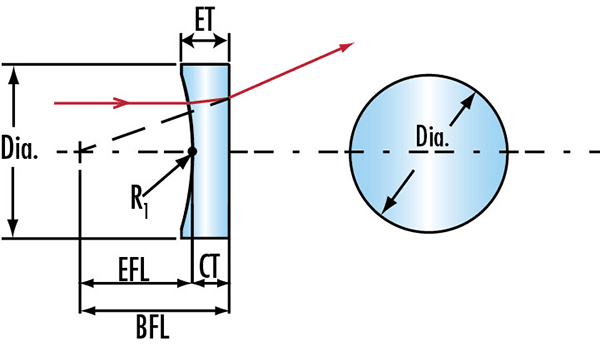#TECHSPEC®組件由愛特蒙特光學設計、指定或製造。進一步瞭解 照明級 PCV(平凹) 柱面透鏡• Cylinder Lenses Ideal for 1 Dimensional Laser Beam Convergence
• Circular and Rectangular Form Factors
• Multiple Coating Options Available

The thin lens approximation for the length of a line generated by a negative cylinder lens is: L = 2 * (r0/f) * (z + f) where L is the line length, r0 is half the beam diameter, z is the projection distance, and -f is the focal length of the lens.×